### 9.5.2.2 The source of prior distributions

Suppose that the Bayesian method has been adopted. The most widespread concern in all Bayesian analyses is the source of the prior distribution. In Section 9.2, this is represented as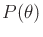(or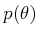), which represents a distribution (or density) over the nature action space. The best way to obtainis by estimating the distribution over numerous independent trials. This brings its definition into alignment with frequentist views. This was possible with Example 9.11, in whichcould be reliably estimated from the frequency of occurrence of letters across numerous pages of text. The distribution could even be adapted to a particular language or theme.

In most applications that use decision theory, however, it is impossible or too costly to perform such experiments. What should be done in this case? If a prior distribution is simply made up,'' then the resulting posterior probabilities may be suspect. In fact, it may be invalid to call them probabilities at all. Sometimes the term subjective probabilities is used in this case. Nevertheless, this is commonly done because there are few other options. One of these options is to resort to frequentist decision theory, but, as mentioned, it does not work with single observations.

Fortunately, as the number of observations increases, the influence of the prior on the Bayesian posterior distributions diminishes. If there is only one observation, or even none as in Formulation 9.3, then the prior becomes very influential. If there is little or no information regarding, the distribution should be designed as carefully as possible. It should also be understood that whatever conclusions are made with this assumption, they are biased by the prior. Suppose this model is used as the basis of a planning approach. You might feel satisfied computing the optimal'' plan, but this notion of optimality could still depend on some arbitrary initial bias due to the assignment of prior values.

If there is no information available, then it seems reasonable thatshould be as uniform as possible over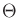. This was referred to by Laplace as the principle of insufficient reason'' . If there is no reason to believe that one element is more likely than another, then they should be assigned equal values. This can also be justified by using Shannon's entropy measure from information theory [49,248,864]. In the discrete case, this is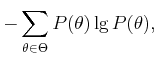(9.89)

and in the continuous case it is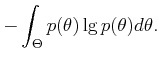(9.90)

This entropy measure was developed in the context of communication systems to estimate the minimum number of bits needed to encode messages delivered through a noisy medium. It generally indicates the amount of uncertainty associated with the distribution. A larger value of entropy implies a greater amount of uncertainty.

It turns out that the entropy function is maximized whenis a uniform distribution, which seems to justify the principle of insufficient reason. This can be considered as a noninformative prior. The idea is even applied quite frequently when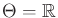, which leads to an improper prior. The density function cannot maintain a constant, nonzero value over all ofbecause its integral would be infinite. Since the decisions made in Section 9.2 do not depend on any normalizing factors, a constant value can be assigned forand the decisions are not affected by the fact that the prior is improper.

The main difficulty with applying the entropy argument in the selection of a prior is thatitself may be chosen in a number of arbitrary ways. Uniform assignments to different choices ofultimately yield different information regarding the priors. Consider the following example.

Example 9..26 (A Problem with Noninformative Priors)   Consider a decision about what activities to do based on the weather. Imagine that there is absolutely no information about what kind of weather is possible. One possible assignment is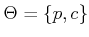, in which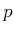means precipitation'' and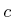means clear.'' Maximizing (9.89) suggests assigning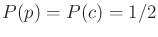.

After thinking more carefully, perhaps we would like to distinguish between different kinds of precipitation. A better set of nature actions would be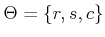, in whichstill means clear,'' but precipitationhas been divided into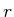for rain'' and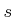for snow.'' Now maximizing (9.89) assigns probability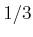to each nature action. This is clearly different from the original assignment. Now that we distinguish between different kinds of precipitation, it seems that precipitation is much more likely to occur. Does our preference to distinguish between different forms of precipitation really affect the weather?Example 9..27 (Noninformitive Priors for Continuous Spaces)   Similar troubles can result in continuous spaces. Recall the parameter estimation problem described in Example 9.12. Suppose instead that the task is to estimate a line based on some data points that were supposed to fall on the line but missed due to noise in the measurement process.

What initial probability density should be assigned to, the set of all lines? Suppose that the line lives in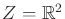. The line equation can be expressed as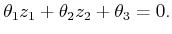(9.91)

The problem is that if the parameter vector,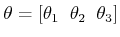, is multiplied by a scalar constant, then the same line is obtained. Thus, even though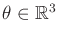, a constraint must be added. Suppose we require that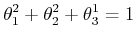(9.92)

and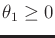. This mostly fixes the problem and ensures that each parameter value corresponds to a unique line (except for some duplicate cases at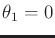, but these can be safely neglected here). Thus, the parameter space is the upper half of a sphere,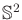. The maximum-entropy prior suggests assigning a uniform probability density to. This may feel like the right thing to do, but this notion of uniformity is biased by the particular constraint applied to the parameter space to ensure uniqueness. There are many other choices. For example, we could replace (9.92) by constraints that force the points to lie on the upper half of the surface of cube, instead of a sphere. A uniform probability density assigned in this new parameter space certainly differs from one over the sphere.

In some settings, there is a natural representation of the parameter space that is invariant to certain transformations. Section 5.1.4 introduced the notion of Haar measure. If the Haar measure is used as a noninformative prior, then a meaningful notion of uniformity may be obtained. For example, suppose that the parameter space is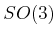. Uniform probability mass over the space of unit quaternions, as suggested in Example 5.14, is an excellent choice for a noninformative prior because it is consistent with the Haar measure, which is invariant to group operations applied to the events. Unfortunately, a Haar measure does not exist for most spaces that arise in practice.9.9Steven M LaValle 2020-08-14